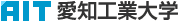日本語
 Please use this identifier to cite or link to this item: http://hdl.handle.net/11133/2022

 Title: C^1級関数f(x)に対する非線形方程式f(x)=0の近似解の収束性について Other Titles: On the convergence of approximate solutions of non-linear equation f(x)=0 for f(x) in C^1-class Authors: 樋口, 功HIGUCHI, Isao Issue Date: 31-Mar-2002 Publisher: 愛知工業大学 Abstract: As the methods of finding the approximate solutions of non-linear equation f (x) = 0, the Newton and the secant methods are well known and are recently recognized again because they are fairly applicable to computer calculation. In these iterative procedures, the curve y = f (x) is approximated by the straight lines and hence the recurrence formulas are relatively simple and the successive calculations are not so difficult. But, in general, the convergence of approximate sequence {x_n} is not necessarily assured. Indeed, in many cases, the sequence {x_n} does not approach to a true solution. For giving the assurrance of the convergence of {x_n} we must determine the suitable extent of starting point x_0. The typical proof of the convergence of {x_n} is done with the help of the so-called principle of contraction mapping under the additional condition that f (x) is in the class C^2 The purpose of this paper is to prove the convergence of {x_n} when f(x) is in C^1 but not necessarily in C^2 And further, the author should like to remark that his method does not ask the help of the principle of contraction mapping and that his new proof is applicable to the case of the more complicated secant method whose approximate sequence is determined by recursion formula of three poins. URI: http://hdl.handle.net/11133/2022 Appears in Collections: 37号

Files in This Item:

File Description SizeFormatDSpace Software Copyright © 2002-2010  Duraspace - Feedback